# Apsis

"Apogee," "Perigee," "Aphelion," "Perihelion" redirect here.When an object (such as the Moon) orbits the Earth, the point along its path that is closest to Earth is called its perigee, and the point farthest from Earth is called its apogee.

When a celestial object is in an elliptical orbit around another body, an apsis (plural apsides) is a point on the orbit at greatest or least distance from the center of attraction, which is generally the center of mass of the system. The point of closest approach is called the periapsis or pericenter, and the point of farthest excursion is called the apoapsis, apapsis, or apocenter. (In technical usage, the words periapsis and apoapsis are preferred over pericenter and apocenter.) A straight line drawn through the periapsis and apoapsis is called the line of apsides, corresponding to the major axis of the ellipse, that is, the line through the longest part of the ellipse.

## Contents

Derivative terms are used to identify the body being orbited. The most common are perigee and apogee, which refer to points on orbits around the Earth, and perihelion and aphelion, which refer to points on orbits around the Sun. The terms perihelion and aphelion are commonly used for orbits of planets in the Solar System, but the same terms are occasionally employed when referring to the nearest and farthest distances of a comet from the Sun. During the Apollo program, the terms pericynthion and apocynthion were used when referring to the spacecraft's orbit around the Moon.

## Etymology

The term apsis is derived from the Latin word apsis, meaning "arch" or "vault," which can be traced back to the Greek word hapsis, meaning "loop" or "arch," from Greek haptein, meaning "fasten together." The prefixes peri and apo can be traced back to Greek terms that mean "near" and "away from," respectively.

In the case of perigee and apogee, the root gee comes from the Greek gê, meaning "Earth." For perihelion and aphelion, the root helion is derived from the Greek helios, meaning "Sun."

As noted above, "-gee" and "-helion" are used for orbits of objects around the Earth and the Sun, respectively. Various additional terms have been coined for orbits around other celestial objects. For example, "-astron" and "-galacticon" are used when the body being orbited is a star or a galaxy, respectively. For orbits around a black hole, physicist Geoffrey A. Landis introduced the terms perimelasma and apomelasma (from the Greek root) in 1998; but later (in 2002), the terms perinigricon and aponigricon (from the Latin) appeared in the scientific literature.

Various terms are listed in the table below. Although some of them are used occasionally, the term -saturnium has rarely been used in the last 50 years. Also, the -gee form is commonly (albeit incorrectly) used as a generic term for "closest or farthest point from a planet," instead of specifically applying to orbits around the Earth.

Body Closest approach Farthest approach
Galaxy Perigalacticon Apogalacticon
Star Periastron Apastron
Black hole Perimelasma/Peribothra/Perinigricon Apomelasma/Apobothra/Aponigricon
Sun Perihelion Aphelion
Mercury Perihermion Apohermion
Venus Pericytherion/Pericytherean/Perikrition Apocytherion/Apocytherean/Apokrition
Earth Perigee Apogee
Moon Periselene/Pericynthion/Perilune Aposelene/Apocynthion/Apolune
Mars Periareion Apoareion
Jupiter Perizene/Perijove Apozene/Apojove
Saturn Perikrone/Perisaturnium Apokrone/Aposaturnium
Uranus Periuranion Apouranion
Neptune Periposeidion Apoposeidion

Given that "peri" and "apo" are derived from Greek, it is considered by some purists more correct to use the Greek form for the body, giving forms such as "-zene" for Jupiter and "-krone" for Saturn. The daunting prospect of having to maintain a different word for every orbitable body in the solar system (and beyond) is the main reason why the generic '-apsis' has become the almost universal norm.

• In the case of the Moon, in practice all three forms are used, albeit very infrequently. The "-cynthion" form is, according to some, reserved for artificial bodies, whereas others reserve "-lune" for an object launched from the Moon and "-cynthion" for an object launched from elsewhere. The "-cynthion" form was the version used in the Apollo Project, following a NASA decision in 1964.
• For Venus, the form "-cytherion" is derived from the commonly used adjective "cytherean;" the alternate form "-krition" (from Kritias, an older name for Aphrodite) has also been suggested.
• For Jupiter, the "-jove" form is occasionally used by astronomers whereas the "-zene" form is never used, like the other pure Greek forms ("-areion" (Mars), "-hermion" (Mercury), "-krone" (Saturn), "-uranion" (Uranus), "-poseidion" (Neptune) and "-hadion" (Pluto)).

## Earth's perihelion and aphelion

The Earth is closest to the Sun in early January and farthest in early July. The relation between perihelion, aphelion and the Earth's seasons changes over a 21,000 year cycle. This anomalistic precession contributes to periodic climate change (related to what are known as Milankovitch cycles).

The day and hour of these events for recent and upcoming years are noted in the table below.

Year Perihelion Aphelion
2007 January 3 20Z July 7 00Z
2008 January 3 00Z July 4 08Z
2009 January 4 15Z July 4 02Z
2010 January 3 00Z July 6 11Z
2011 January 3 19Z July 4 15Z
2012 January 5 00Z July 5 03Z
2013 January 2 05Z July 5 15Z
2014 January 4 12Z July 4 00Z
2015 January 4 07Z July 6 19Z
2016 January 2 23Z July 4 16Z

## Mathematical formulae

The following mathematical formulae characterize the periapsis and apoapsis of an orbit:

• Periapsis: maximum speed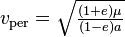$v_\mathrm{per} = \sqrt{ \tfrac{(1+e)\mu}{(1-e)a} } \,$ at minimum (periapsis) distance$r_\mathrm{per}=(1-e)a\!\,$
• Apoapsis: minimum speed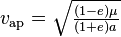$v_\mathrm{ap} = \sqrt{ \tfrac{(1-e)\mu}{(1+e)a} } \,$ at maximum (apoapsis) distance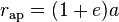$r_\mathrm{ap}=(1+e)a\!\,$

while, in accordance with Kepler's laws of planetary motion (conservation of angular momentum) and the conservation of energy, these quantities are constant for a given orbit:

• Specific relative angular momentum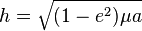$h = \sqrt{(1-e^2)\mu a}$
• Specific orbital energy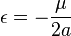$\epsilon=-\frac{\mu}{2a}$

where:

•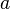$a\!\,$ is the semi-major axis
•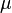$\mu\!\,$ is the standard gravitational parameter
•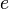$e\!\,$ is the eccentricity, defined as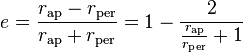$e=\frac{r_\mathrm{ap}-r_\mathrm{per}}{r_\mathrm{ap}+r_\mathrm{per}}=1-\frac{2}{\frac{r_\mathrm{ap}}{r_\mathrm{per}}+1}$

Note that for conversion from heights above the surface to distances between an orbit and its primary, the radius of the central body has to be added, and conversely.

The arithmetic mean of the two limiting distances is the length of the semi-major axis$a\!\,$. The geometric mean of the two distances is the length of the semi-minor axis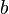$b\!\,$.

The geometric mean of the two limiting speeds is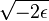$\sqrt{-2\epsilon}$, the speed corresponding to a kinetic energy which, at any position of the orbit, added to the existing kinetic energy, would allow the orbiting body to escape (the square root of the product of the two speeds is the local escape velocity).